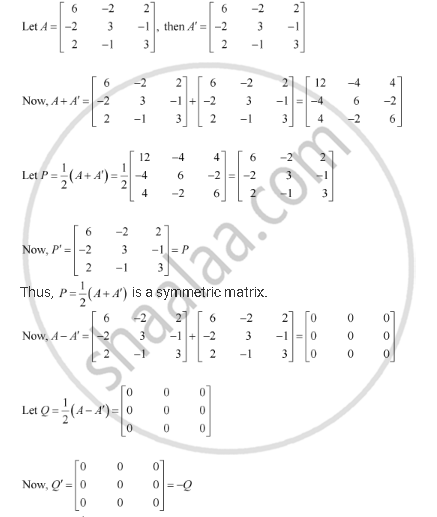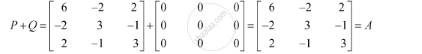Share

# Express the Matrices as the Sum of a Symmetric and a Skew Symmetric Matrix: [(6, -2,2),(-2,3,-1),(2,-1,3)] - CBSE (Science) Class 12 - Mathematics

ConceptSymmetric and Skew Symmetric Matrices

#### Question

Express the matrices as the sum of a symmetric and a skew symmetric matrix:

[(6, -2,2),(-2,3,-1),(2,-1,3)]

#### SolutionThus, Q =1/2(A -A') is a skew-symmetric matrix.

Representing A as the sum of P and Q:Is there an error in this question or solution?

#### Video TutorialsVIEW ALL 

Solution Express the Matrices as the Sum of a Symmetric and a Skew Symmetric Matrix: [(6, -2,2),(-2,3,-1),(2,-1,3)] Concept: Symmetric and Skew Symmetric Matrices.
S